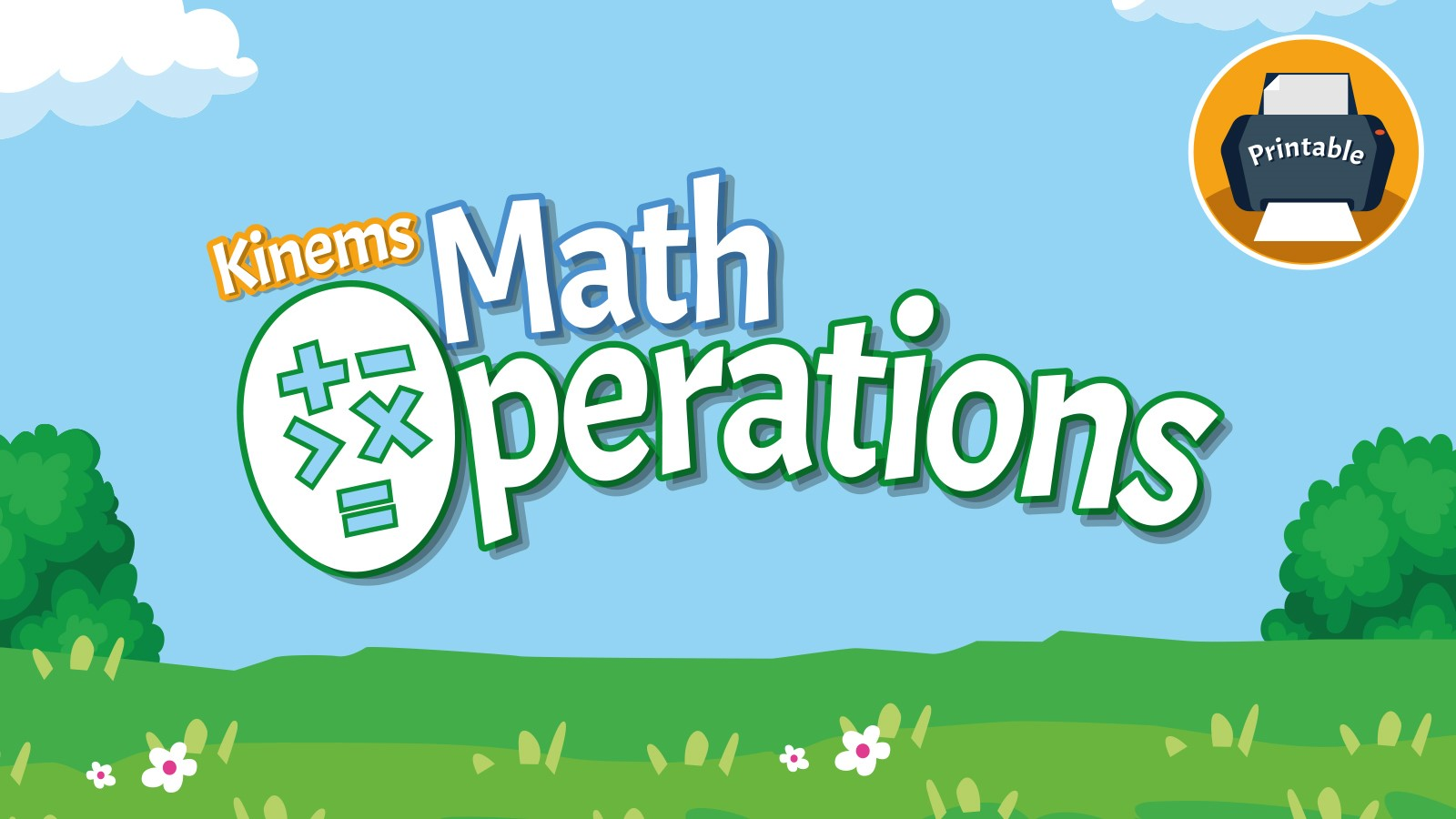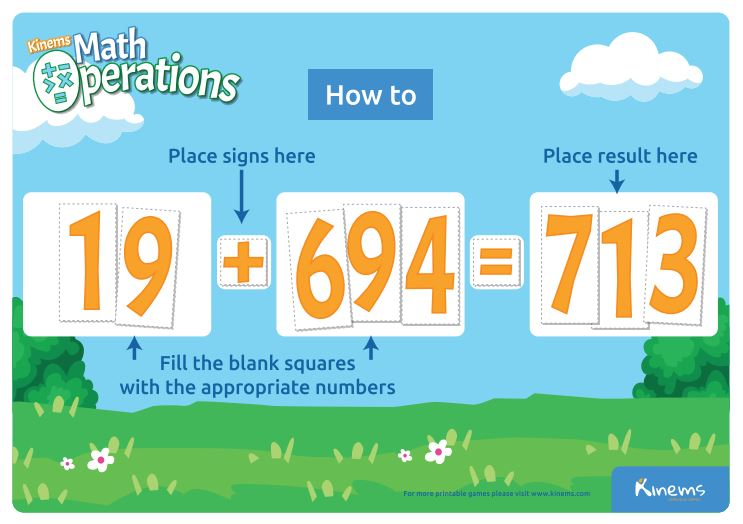### Free Printable Board Game | Kinems Math OperationsAre you looking for a fun and engaging way to teach math operations to young children?

This is the right place for you!

Kinems Math Operations Board Game helps students to develop their math computation skills by practicing addition, subtraction, multiplication, division with whole numbers up to 100.

Math fluency in solving computation teaches critical thinking skills. Math teaches order and logic. A mathematical equation has a predictable outcome. To solve it you learn the precise steps which must be followed in order to solve the problem.

Children will need to learn to add and subtract within 20, demonstrating fluency for addition and subtraction within 10. Use strategies such as counting; making ten (e.g., 8 + 6 = 8 + 2 + 4 = 10 + 4 = 14); decomposing a number leading to a ten (e.g., 13 - 4 = 13 - 3 - 1 = 10 - 1 = 9); using the relationship between addition and subtraction (e.g., knowing that 8 + 4 = 12, one knows 2 - 8 = 4); and creating equivalents. Older elementary students will need to be fluent and memorize math facts in multiplication and division as well.#### How to play

Game 1: Solve the operations

Give your children a paper with a list of math problems using operations they need to solve according to their grade level. Ask them to use the math operation cards and cut out numbers to find the solution on the template and then solve it on the assigned sheet by writing in the answers.

Give your children specific numbers and operation symbols from the cutouts and ask them to make up as many operations as possible. Encourage them to keep notes of the operations that they created on a piece of paper. Then, together check the operations and support them further to solve correctly, if needed.

Game 3: Find the missing operation symbol

Work together and provide them with an operation where the operation symbol will be missing. For example: 5 _ 5 = 10. Ask them to find and fill the blank square with the correct sign to complete the operation.

Game 3: Find the missing number

Work together and provide them with an operation where this time the number will be missing. For example: 5 + _ = 10. Ask them to find and fill the blank with the missing number to complete the operation.

Tip: Use the heart cut-outs from the Kinems Board Game Yummy Pairs as a reward for their correct answers. How many little heart cards can they get?

#### More ideas for math in daily life

• When making a shopping list, have your child help determine how much of each item is needed to feed the family. Use real world word problems. If we want everyone to have two pieces of chicken, how many do we need to buy?
• When cooking, have your child read the recipe to follow along and use the ingredients as word problems to solve with computation.
• If you have dice in the house, you can play games by throwing the dice and using the numbers to multiply, add, subtract or divide. You can make it a competition if you have family members also playing. The first person to solve the equation, wins.
• If you have a deck of cards, you can decide to make the face cards equal 1 or 10. Decide on the operation ahead of time. Say you decide it is an addition. Deal out 5 cards to each player. Each player puts down one card on the table. Say one player put down a 2 and another player put down a 7. The first player to say 9, wins both cards. The winner is the player that ends up with the most cards.
• Use Skittles, cheerios, m&ms or goldfish as counter manipulatives to teach young children early math computation. Use them to add and subtract. You can use the sheets in this game and the operations signs while using these real world and yummy counters. Accurate operations can be eaten!
• Go into the sock drawer. Determine how many socks there are and how long you can go before having to do laundry. You wear two every day. When do you need to wash your socks?
• Count how many windows are in the house. How many are in each room? How many rooms are there? Make a floor plan. How many doors are there?
• Get an empty jar. Fill it with jelly beans, regular dry beans or any small items that can be easily counted. Ask everyone in the house to guess how many are in the jar. Write down the answers and their name. Then when everyone has guessed, open the jar and ask your child to count them. Put the beans or items in piles of 10 so you are teaching counting by 10s in real life!